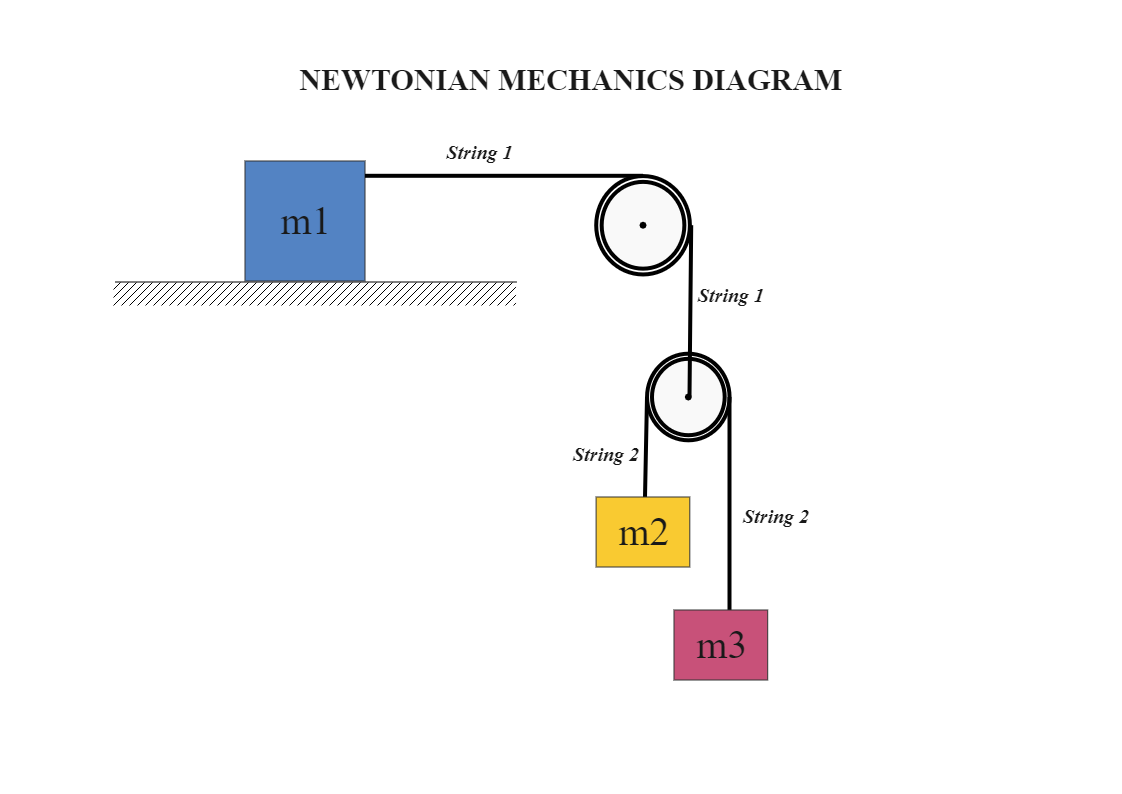Template Community / NEWTONIAN MECHANICS DIAGRAM

# NEWTONIAN MECHANICS DIAGRAMeasy diagrams
Published on 2020-09-16
Edit OnlineThis diagram represents the Newtonian mechanics diagrams. The tension on m2 and m3 due to the string is represented effectively. The determination of the equations of motion for an n-body system, where individual forces act on the individual mass of the n-body system, is a fundamental goal of mechanics. To compute the instantaneous spatial positions, velocities, and accelerations for each mass, Newton's second-order equation of motion must be solved. Use EdrawMax to create this diagram and other 280+ types of diagrams. You can find more diagram templates from the template gallery, and you can also start creating graphs with 26,000+ vector symbols.
Tag
science diagram
mechanics diagram
Report
6
257PostRecommended Templates